Scattering and tunnelling

Start this free course now. Just create an account and sign in. Enrol and complete the course for a free statement of participation or digital badge if available.

Free course

# 3.4 Probability currents

The expressions we have derived for reflection and transmission coefficients were based on the assumption that the intensity of a beam is the product of the speed of its particles and their linear number density. This assumption seems very natural from the viewpoint of classical physics, but we should always be wary about carrying over classical ideas into quantum physics. In this section we shall establish a general quantum-mechanical formula for the beam intensity. The formula will be consistent with the assumptions made so far, but is also more general, applying in regions where a classical beam would not exist and for localised wave packets as well as steady beams.

At the heart of our analysis lies the idea that matter is conserved. Neglecting relativistic processes in which particles can be created or destroyed, the total number of particles remains fixed. This is built deep into the formalism of quantum mechanics: if the wave function describing a particle is normalised now, it will remain normalised forever because particles do not simply disappear. The conservation of particles applies locally as well as globally, so if the number of particles in a small region changes, this must be due to particles entering or leaving the region by crossing its boundaries. We shall now express this idea in mathematical terms.

Let us first consider the one-dimensional flow of a fluid along the x-axis. At each point, we define a fluid current jx(x, t) that represents the rate of flow of fluid particles along the x-axis. If the fluid is compressible, like air, this fluid current may vary in space and time.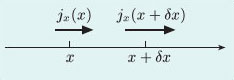Figure 11 Any change in the number of particles in a small region is due to fluid currents that carry particles into or out of the region

Figure 11, above, shows a small one-dimensional region between x and x + δx. The number of particles in this region can be written as n(x, t) δx, where n(x, t) is the linear number density of particles. The change in the number of particles in the region during a small time interval δt is then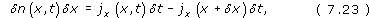where, for flow in the positive x-direction, the first term on the right-hand side represents the number of particles entering the region from the left and the second term represents the number of particles leaving the region to the right. Rearranging Equation 7.23 gives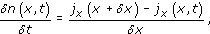and, on taking the limit as δx and δt tend to zero we see that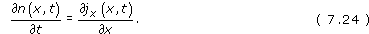This result is called the equation of continuity in one dimension. With a little care, it can be extended to quantum mechanics.

For a single-particle wave packet in quantum mechanics, the flowing quantity is probability density. This is evident from images of wave packets as ‘blobs’ of moving probability density (e.g. Figure 6). Now we know that probability density is represented in quantum mechanics by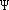*, so we should be able to construct the appropriate equation of continuity by examining the time derivative of this quantity.

Obviously, we have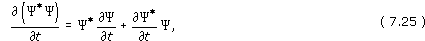where Schrödinger's equation dictates that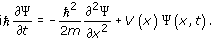Dividing through by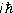, the rate of change of the wave function is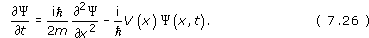Substituting this equation, and its complex conjugate, into Equation 7.25, we then obtain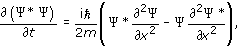(since the potential energy function V(x) is real and cancels out), and a further manipulation gives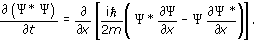This equation can be written in the form of an equation of continuity: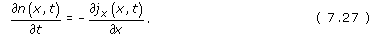provided that we interpret n(x, t) as the probability density*, with a corresponding current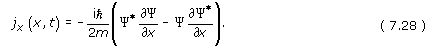In the one-dimensional situations we are considering, jx(x, t) is called the probability current. In one dimension, the probability density n =*is a probability per unit length, and therefore has the dimensions of [L]−1. It follows from Equation 7.27 that the probability current has dimensions of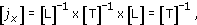and therefore has SI unit ‘per second’, as expected for a current of particles.

These ideas can be readily extended to steady beams of particles. Snapshots of a steady beam would not reveal any changes from one moment to the next but the beam nevertheless carries a steady flow of particles, just as a steadily flowing river carries a current of water. For a particle beam,*represents the linear number density of particles and the probability current is the rate of flow of particles in the positive x-direction. For a steady beam, described by a stationary-state wave function,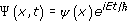, the time-dependent phase factors cancel out in Equation 7.28, and the probability current can be written more simply as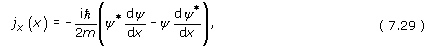which is independent of time. It is important to realise that jx is a signed quantity; it is positive for a beam travelling in the positive x-direction, and negative for a beam travelling in the negative x-direction. This is unlike the beam intensity j introduced earlier, which is always positive. It is natural to define the beam intensity of a steady beam to be the magnitude of the probability current: j = |jx|. It is this definition that gives us a way of calculating beam intensities without making unwarranted classical assumptions.

In fact, each beam intensity calculated using Equation 7.29 turns out to be precisely what we have always assumed – the product of a particle speed and a linear number density – as you can check by tackling Exercise 7 below. So our analysis adds rigour, but contains no surprises. However, the really significant feature of Equation 7.28 is its generality; it applies to single-particle wave packets as well as to steady beams, and (as you will see later) it will also apply in cases of tunnelling, where a classical beam does not exist.

## Exercise 6

Show that jx(x, t) as defined in Equation 7.28 is a real quantity.

One way of showing that a quantity is real is to show that it is equal to its own complex conjugate. Taking the complex conjugate of each factor in jx, we obtain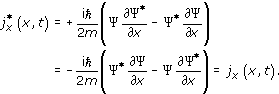Since j*x = jx, we conclude that jx is real.

## Exercise 7

Using the solutions to the Schrödinger equation that were obtained in the stationary-state approach to scattering from a finite square step, evaluate the probability current in the regions x > 0 and x ≤ 0. Interpret your results in terms of the beam intensities in these two regions.

In the region x > 0,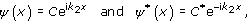so Equation 7.29 gives the probability current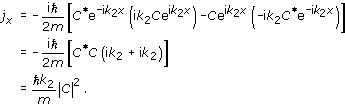In the region x ≤ 0,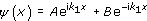and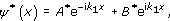so Equation 7.29 gives the probability current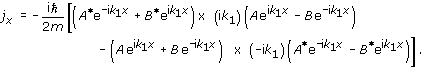Simplifying this expression, we obtain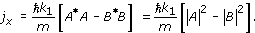This can be interpreted as the sum of an incident probability current,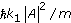, and a reflected probability current,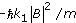. These two contributions have opposite signs because they flow in opposite directions. Note that, in each region, the probability currents are consistent with the incident, reflected and transmitted beam intensities assumed earlier (and now justified).

SM358_1